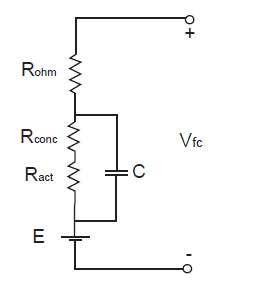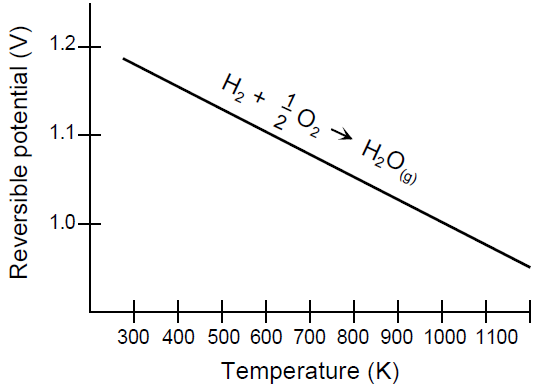CATEGORIES:

# Fuel cells performance

Fuel cell uses gases to produce electrical energy; hence, we are interested in its electrical characteristics. Schematically fuel cell’s equivalent circuit could be represented as shown below, in the figure 4.Fig. 4 Fuel Cell equivalent circuit

The actual cell potential is decreased from its ideal potential because of several types ofirreversible losses, as shown in Figure 4. These losses are often referred to as polarization,overpotential or overvoltage, though only the ohmic losses actually behave as a resistance.

Multiple phenomena contribute to irreversible losses in an actual fuel cell:

• Activation-related losses. These stem from the activation energy of the electrochemicalreactions at the electrodes. These losses depend on the reactions at hand, the electro-catalystmaterial and microstructure, reactant activities (and hence utilization), and weakly on currentdensity. 

• Ohmic losses. Ohmic losses are caused by ionic resistance in the electrolyte and electrodes,electronic resistance in the electrodes, current collectors and interconnects, and contactresistances. Ohmic losses are proportional to the current density, depend on materialsselection and stack geometry, and on temperature. 

• Mass-transport-related losses. These are a result of finite mass transport limitations rates ofthe reactants and depend strongly on the current density, reactant activity, and electrodestructure. 

In the V-I diagram, especially for low-temperature fuel cells, the effectsof the three loss categories are often easy to distinguish, as illustrated in Figure 5.Fig. 5 - Voltage drops in Fuel Cell causedby different reasons. 

Figure 6 shows the relation of cell’s voltage to itstemperature. Because the figure shows the potential ofhigher temperature cells, the ideal potential corresponds to a reaction where the water product isin a gaseous state (i.e., E0 is 1.18 volts).Fig. 6 - H2/O2 Fuel Cell Ideal Potential as a Function of Temperature.

As for efficiency of a unit cell, it is related to voltage of unit fuel cell and can be depicted the way below (figure 7, a). Total efficiency of a system is shown in figure 7, b. ICE-efficiency diagram is added to comparison. Fuel cell has better efficiency in every load conditions (0-100%) but the highest is at about 10-15 % load. Operating window is between 15 and 30 % and it is approximately 2 times better than efficiency of ICE.Fig. 7 – Efficiency of a unit cell (a) and total efficiency of a system in compare with

ICE-system (b)

Date: 2014-12-29; view: 652

 <== previous page | next page ==> Automotive Fuel Cell Systems | Fuel cells applications
doclecture.net - lectures - 2014-2019 year. Copyright infringement or personal data (0.002 sec.)